### Intro to Data Science

Week 6: Regression vs. Classification

March 18, 2019

###### Today's Agenda
1. Regression vs. Classification
2. Logistic Regression
3. Measuring Performance for Classification Models
4. Midterm Recap
###### Week 5 Recap
1. Linear Regression
2. Assumptions for Linear Models
3. Measuring Performance for Linear Models
###### Data Science Models###### Key Metrics: Linear Regression
1. R-squared
3. Coefficients
4. P-values
R-Squared: Share of the target variation that is explained by the model.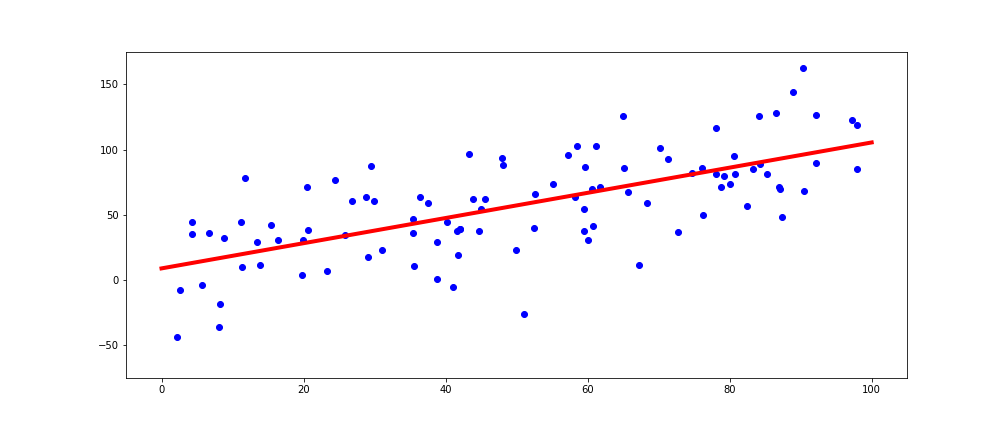###### Generalized Linear Models

A flexible generalization of ordinary linear regression that allows for response variables that have error distribution models other than a normal distribution.

###### Generalized Linear Models###### Regression vs. Classification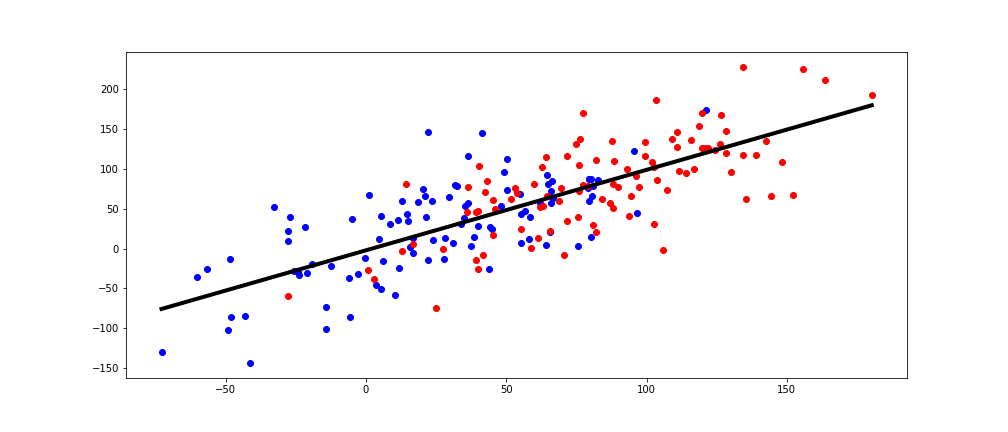Is this a good forecast?

Regression analysis estimates the conditional expectation of the dependent variable given the independent variables.

Classification is the problem of identifying to which of a set of categories a new observation belongs.

###### Regression vs. Classification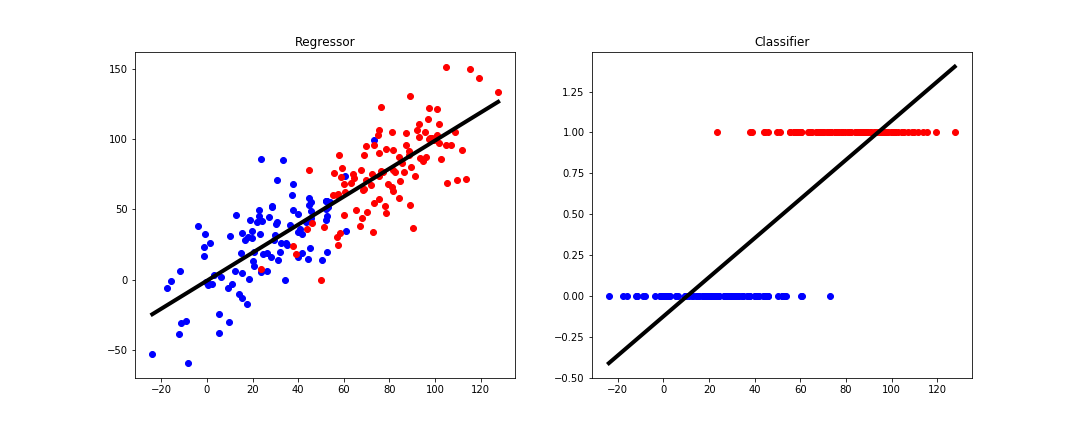###### Logistic Regression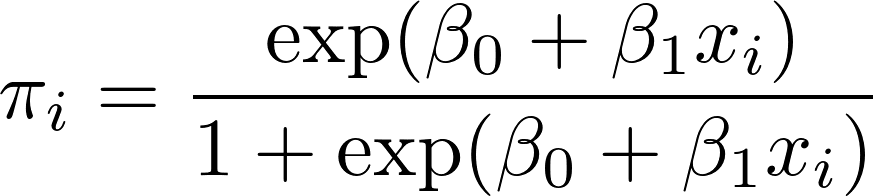###### Logistic Regression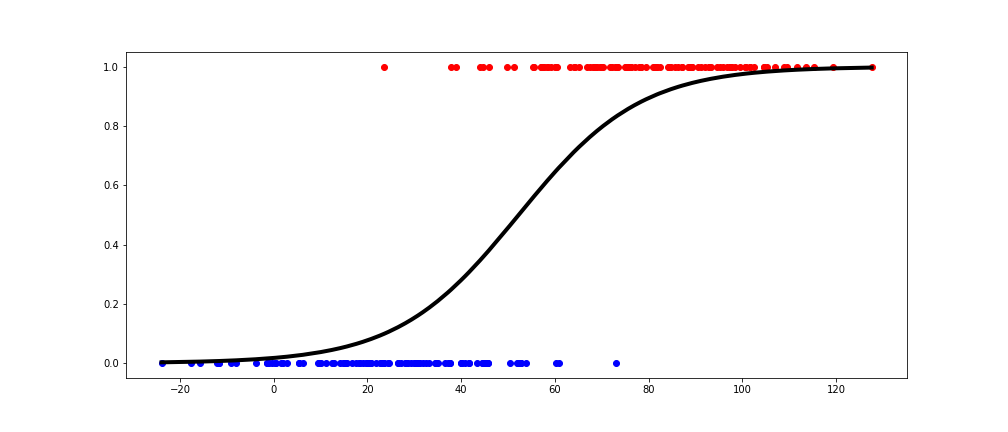###### Regression vs. Classification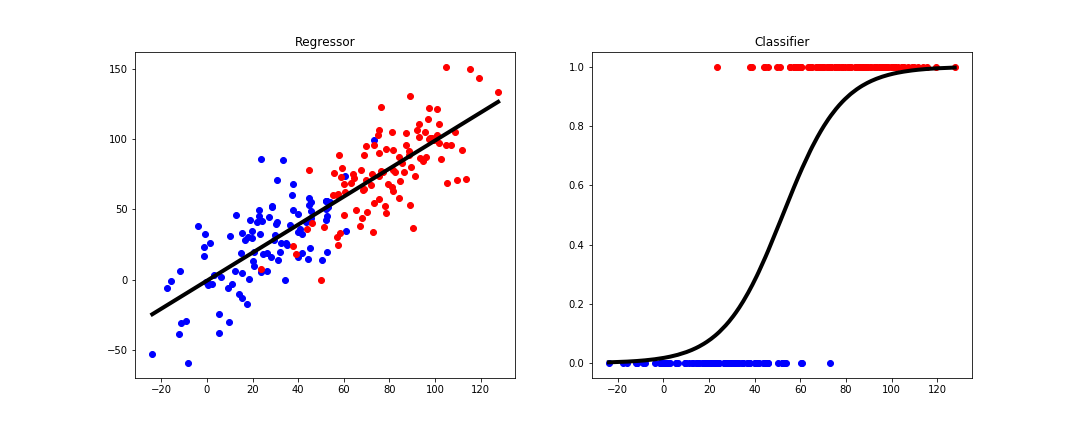###### Logistic Regression Output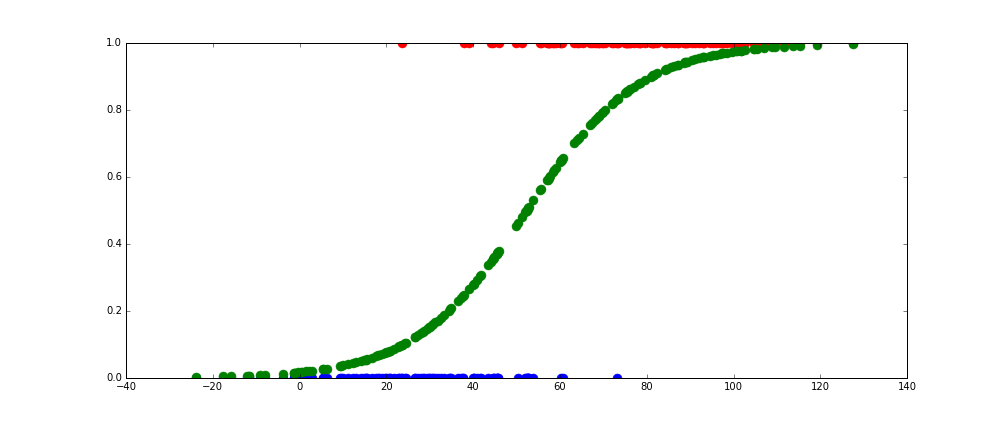Logisitic regression and many other classification models output a continuous value between 0 and 1.

###### Measuring Classification Performance
1. Confusion Matrix
2. Precision
3. Recall
4. Accuracy
(as explained by the zombie apocalypse)
###### Confusion Matrix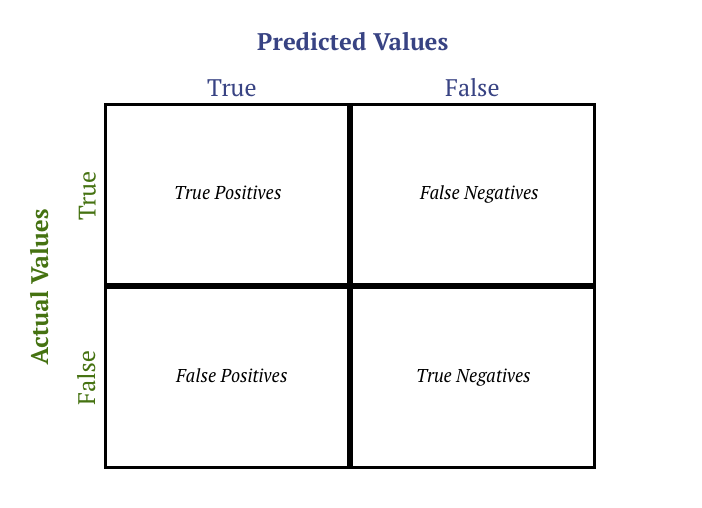###### Precision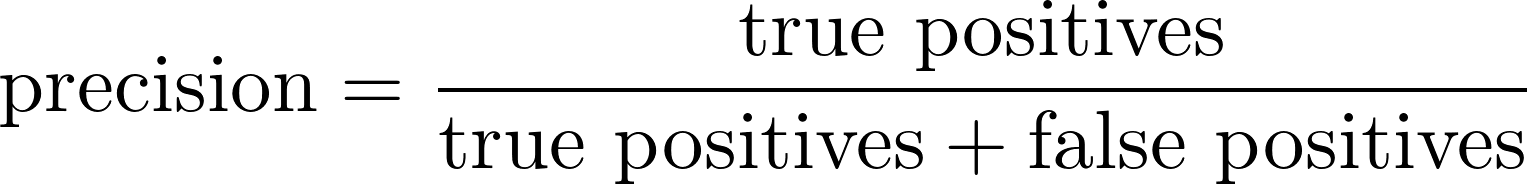zombie apocalypse use case: you're hunting zombies, and you need to kill as many zombies as possible without killing any humans

###### Recall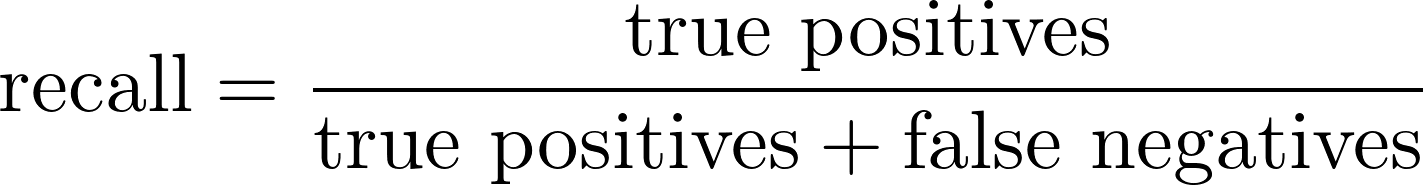zombie apocalypse use case: you discover a cure for zombies, but can only apply it k infected people

###### Accuracy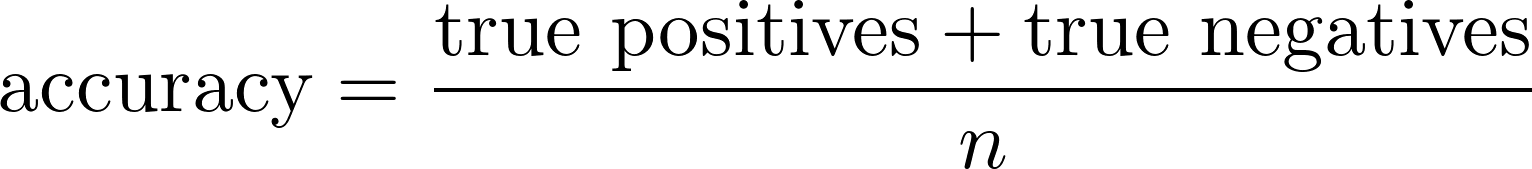zombie apocalypse use case: zombies have infected roughly half the population, and you're throwing them a party. you are putting together an invite list and want to make sure you invite an equal amount of zombies and humans.

###### Midterm: March 25, 6:30pm
• 90 Minute Written Exam
• No computer needed, closed book, closed notes
• Part I: Multiple Choice
Review
###### Week 2: Where to Find Data
• The data science lifecycle.
• Structured vs unstructured data.
• Common sources of data.
• Common ways to access data.
###### Week 3: Processing and Cleaning Data
• Elements of the ETL Process
• Processing Tools
• Data Cleaning Considerations for Data Scientists
• Missing Value
• Handling Outliers
• Normalizing Data
###### Week 4: Statistics and the Stories We Tell Ourselves
• Types of Data
• Useful Statistical Distribution
• Important Summary Statistics
• Independence
• Key Theorems
###### Week 5: Intro to Linear Models
• What Makes Linear Regression Linear
• Assumptions for Linear Models
• Measuring Performance for Linear Models
###### Week 6: Regression vs Classification
• Regression vs. Classification
• Logistic Regression
• Measuring Performance for Classification Models